To study reflection in concave mirror

## Objective:

To study reflection in concave mirror and observe image formations for different positions of the object.

## Related terms:

1. Reflection:
Whenever light, travelling in one medium, comes in contact with surface of another medium, a part of it is returned into the first medium. The phenomenon of returning of light into first medium is known as reflection of light.
2. Concave mirror
A concave mirror is that spherical mirror in which the reflection of light takes place at the concave surface i.e. bent-in surface.
3. Pole
It is the center of the reflecting surface of the concave mirror also called vertex of mirror, generally denoted by letter ‘P’.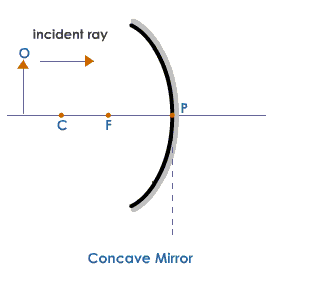4. Center of curvature
It is the center of that sphere of which the concave mirror forms a part, denoted by letter ‘C’.
5. Principal axis
The straight line passing through the center of curvature and pole of  concave mirror is called its principal axis.
6. Principal focus:
A beam of light incident parallel to the principal axis, after reflection from the spherical mirror, either actually converges to or appears to diverge from a fixed point on the principal axis. The fixed point is called the ‘Principal focus’, denoted by letter 'F'.
7. Laws of Reflection:
1)    The angle of incidence is equal to the angle of reflection.
2)    The incident ray, the reflected ray and the normal at the point of incidence, all lie in the same plane.
8. Types of images:
1)    Real image: If the reflected rays actually meet at a point, then the image formed is real. It can be obtained on screen.
2)    Virtual Image: If the reflected rays do not actually meet at a point but appear to diverge from a point, then the image formed is virtual.

Images of an object, formed by a concave mirror, when the object is placed at various positions: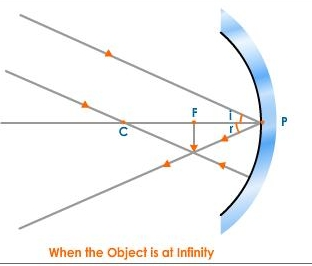When object is at infinity.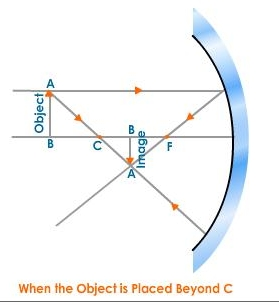When object is beyond centre of curvature('C').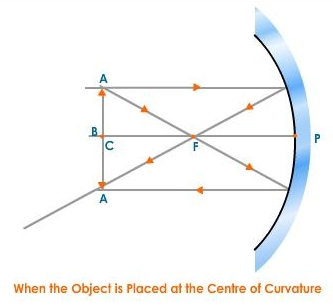When object is at centre of curvature ('C').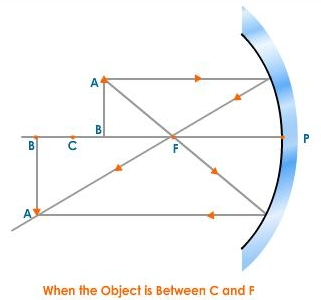When object is between 'C' and 'F'.When object is at focus 'F'.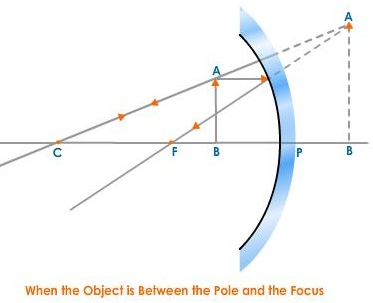When object is between 'P' and 'F'.

Image source: http://www.tutorvista.com/content/science/science-ii/reflection-light/formation-concave-mirror.php

Cite this Simulator: